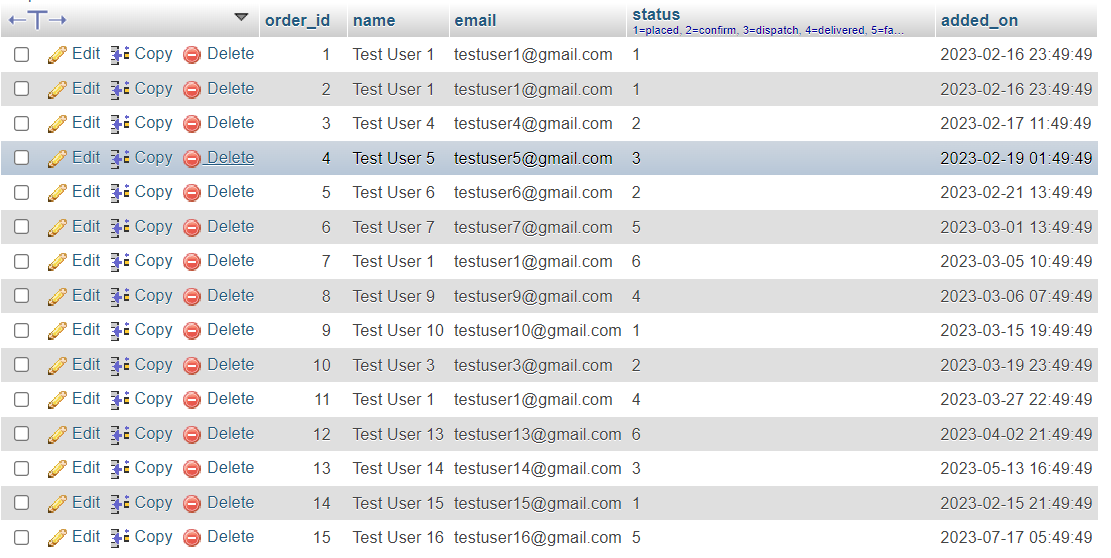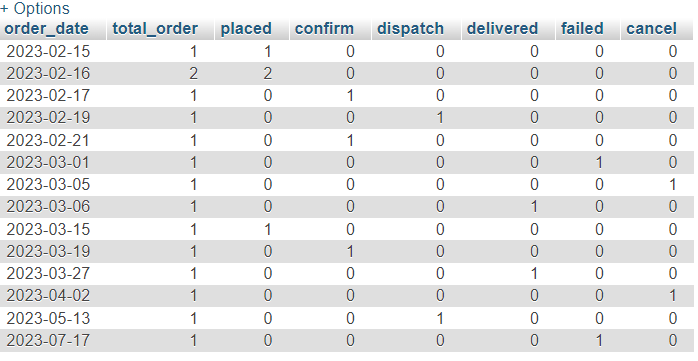# SQL - Total Orders Count According to Date## SQL - Total Orders Count According to Date

##### Published Feb 19,2023 by Kailash Singh

0 Comment     46 Views

In this tutorial, we are going to teach you, how to show order count according to dates using SQL.

Example: You have a table of order records. Inside the table you have different types of status records. LIKE: placed, confirm, dispatch, delivered, failed and cancel.

Table view:SQL Query:

In this query, we are using DATE() function. Because, the DATE() function returns a value that represents the date part of the datetime_expression. It returns a date value in the format 'YYYY-MM-DD'.

For example, if the datetime_expression is '2023-02-19 15:30:45', the DATE() function would return '2023-02-19'.

``````SELECT
count(order_id) as total_order,
sum(if(status=1,1,0)) as placed,
sum(if(status=2,1,0)) as confirm,
sum(if(status=3,1,0)) as dispatch,
sum(if(status=4,1,0)) as delivered,
sum(if(status=5,1,0)) as failed,
sum(if(status=6,1,0)) as cancel
FROM
`orders`
Group By

Result: